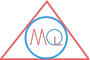# Polygon and its Types

## Introduction

Polygon term originates from the two Greek words “Poly” and “Gon”, where “Poly” stands for “many” and “Gon” stands for angle. So the complete word “Polygon” deals with shapes having many angles.

Interestingly, number of angles in a shape is always equal to the number of edges or sides it may have. In short, we can say a polygon is a shape with many sides or angles.

So, what are the various types of polygons in geometry and how they are made?

Not to go so far, we use curves to make various shapes in geometry. So, to draw all polygons we use curves. Polygon has its angels, edges, vertices and diagonals. We can visualise them and see how many are they in any polygon.

## What is a Curve?

Curve is a plane figure which is made by joining a number of points without lifting a pencil from paper and without retracing any portion of drawing.

Moreover, a curve which do not intersect itself is called open curve and on the other hand, a curve which intersect itself is called closed curve.

Curve in geometry## What is a Polygon in Geometry?

Polygon is a closed curve which is made up of only finite number of line segments and any two line segments do not intersect each other except at their end points. It is a two dimensional figure in geometry.

The line segments of a polygon are called sides or edges and end points of the line segments are called its vertices or corners.

Different types of polygonAny two sides of a polygon with a common end point are called adjacent sides of the polygon.

The end points of a side of a polygon are called adjacent vertices.

## What is a Diagonal of polygon?

A diagonal is a line segment which is formed by joining two vertices which are not adjacent.

Example

AC is a diagonal. BD is a diagonal.

Diagonals## Types of polygon

### Convex polygon

A polygon is said to be a convex polygon, if its diagonals completely lie inside of it. Its all interior angles are less than 180 degree.

Convex Polygon### Concave polygon

A polygon is said to be a concave polygon, if its diagonals completely lie outside of it. Its one or more than one interior angle is more than 180 degree.

Concave Polygon### Regular polygon

A regular polygon is a polygon whose all sides and all angles are equal. In other words we can say, it is both equilateral and equiangular.

Example

Square is a regular polygon as its sides are equal and all angles are equal.

Regular PolygonFormula

Interior angle of regular polygon

$$= (n-2)*180^o$$

or

$$= (n-2)*\pi \; radian$$

### Irregular polygon

Polygon which is not a regular polygon, i.e. if it is not equiangular and not equilateral is called irregular polygon.

Example

Rectangle is a irregular polygon.

Irregular Polygon### Simple polygon

It is a flat shape consisting of straight and non intersecting line segments that are joined to form a single close path. A Simple Polygon does not cross over itself. It is also called Jordan Polygon.

All convex polygons are simple.

Simple Polygon## Classification of polygon based on number of sides

Number of sides of polygonName of polygonNumber of angles of polygon
3Triangle3
5Pentagon5
6Hexagon6
7Heptagon7
8Octagon8
9Nonagon9
10Decagon10

### Q) What is a polygon?

A closed plane figure formed by line segments and two line segments which intersect at only their end points.

### Q) What is sum of angles of polygon?

The sum of angles of polygon with n sides is (n-2)1800.

### Q) What is the sum of exterior angles of polygon?

Sum of exterior angles of polygon is 3600.

### Q) Is equilateral triangle a regular polygon?

Yes, equilateral triangle is a regular polygon as its all sides are equal and all its angles are equal.

### Q) Is rectangle a regular polygon?

No, rectangle is not a regular polygon as its angles are equal but all sides are not equal.

## Solved Examples

### 1) Find the number of sides of polygon if each interior angle is 1400.

Solution

Let number of sides of polygon = n

Each interior angle = $$\frac{(n-2)180^o}{n}$$

140 = $$\frac{(n-2)180}{n}$$

140n = 180n - 360

360 = 180n - 140n

360 = 40n

$$\frac{360}{40}$$ = n

n = 9

### 2) Find the number of sides of regular polygon if each exterior angle is 400

Solution

Sum of all exterior angle = 3600

Each exterior angle = 400

Number of exterior angles = $$\frac{360}{40}$$

Number of exterior angles = 9

∴ Number of sides of polygon = 9

### 3) If each interior angle of regular polygon is 1500 then find its exterior angle.

Solution

Each interior angle = 1500.

We know that, interior angles + exterior angles = 1800

1500 + exterior angles = 1800

exterior angles = 1800 - 1500

exterior angles = 300

### 3) The ratio between exterior angle and interior angle of a regular polygon is 5:1. Find the number of sides of polygon.

Solution

Let exterior angle and interior angle be x and 5x respectively.

Also, interior angles + exterior angles = 1800

5x + x = 1800

6x = 1800

x = $$\frac{180}{6}$$

x = 300

∴ exterior angle = 300

interior angle = 5x = 5 x 300

i.e. interior angle = 1500

## Multiple Choice Questions

a) hexagon

b) decagon

c) pentagon

d) nonagon

a) hexagon

b) heptagon

c) octogon

d) decagon

a) of 900

b) of 1800

c) < 900

d) < 1800

a) < 900

b) < 1800

c) > 900

d) > 1800

### 5) A polygon is said to be regular polygon, if all of its

a) interior angles are equal

b) exterior angles are equal

c) sides are equal

d) all of these

a) 900

b) 1800

c) 2700

d) 3600